0

# TS基础了解一

1、定义

2、特点

3、使用

4、TS基础类型

5、TS引用数据类型

6、类型推论

7、类型别名与联合类型

8、类型断言

1、定义：

（1）TypeScript，简称为TS，是JS的超级。

（2）TS是可以直接运行的（比如使用ts-node），最终编译为纯的JS运行在任意的平台之上，是开源的。

2、特点

``````// 举例
console.log(1 + '1');
// 打印出字符串 '11', ts和js都不会报错

print(1 + '1')
Python 是强类型会报错
# TypeError: unsupported operand type(s) for +: 'int' and 'str'
``````

``````       （2）TS 的类型系统导致它非常适用于大型项目

（3）坚持与 ECMAScript 标准同步发展
``````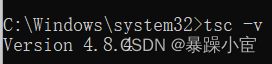3、使用

（1）安装node

（2）全局安装typescript

``````npm i -g typescript
``````

（3）查看版本

``````     tsc -v
``````

（4）创建tsconfig.js文件

``````     tsc  --init
``````

（1）创建index.ts

``````let name1:string="下课";
console.log(name1);
``````

（2）进入index.ts文件，打开cmd，执行命令。

``````     tsc index.ts
``````

（1）安装全局的ts-node模块

``````      npm install -g ts-node
``````

（2）cmd输入指令就可以直接运行

``````      ts-node index.ts
``````

4、TS基础类型

（1）与js相同，使用var、let或const等。

（2）声明变量指定类型(任意类型都可)，这样的变量赋值时只能使用指定类型的值，以达到强类型语言变量的特点及其优点

``````//string
var names:string = '小王'
//number
var age:number = 23;
//bollean
let flag:boolean = true;
flag = 23;//编译出错
``````

（1）布尔值（Boolean）

``````let d:boolean;

d=100<200

console.log(d)

// 编译通过
// 后面约定，未强调编译错误的代码片段，默认为编译通过

let isLogin2: boolean = new Boolean(1);
//编译报错
//使用构造函数 Boolean 创造的对象不是布尔值
``````

（2）数字（number）

``````let n1: number = 6;
let n2: number = 0xf00d;// ES6 中的十六进制表示法,会被编译为十进制数字
let n3: number = 0b1010;// ES6 中的二进制表示法,会被编译为十进制数字
let n4: number = 0o744;// ES6 中的八进制表示法,会被编译为十进制数字
let n5: number = NaN;
let n6: number = Infinity;
``````

（3）字符串

``````let myName: string = 'karen';
let myAge: number = 25;

// 模板字符串
let str: string = `Hello, my name is \${myName}.
I'll be \${myAge + 1} years old next month.`;

let b:String; // 这个跟string不一样 大写的是函数
``````

（4）undefined和null两者各自有自己的类型undefined和null。 和 void相似，用处不大。

（5）void

``````let a:void
function fn():void{

}
function fn2():number{

return 100
}
fn2()
``````

（6）never

never类型表示的是那些永不存在的值的类型。 例如， never类型是那些总是会抛出异常或根本就不会有返回值的函数表达式或箭头函数表达式的返回值类型

``````// 返回never的函数必须存在无法达到的终点
function error(message: string): never {
throw new Error(message);
}

// 推断的返回值类型为never
function fail() {
return error("Something failed");
}
``````

（7）Any

any类型的变量 可以赋值任何类型，任何类型都是any类型的子类型

``````let myname: any;
myname='123';
myname=567;
myname=true;
console.log(myname);
``````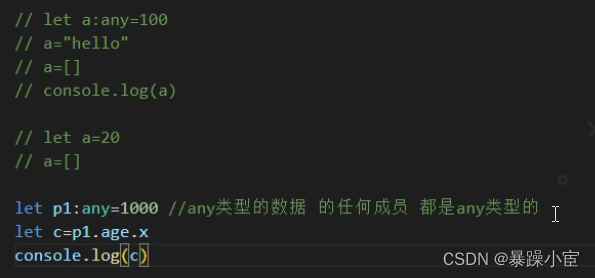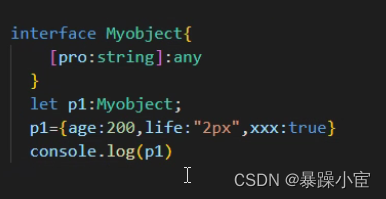（8）数组

TS像JS一样可以操作数组元素。 有两种方式可以定义数组。

``````//第一种方法
let arr:number[];
arr=[10,20,30]
arr=["hello",100]

//第二种方法
let arr:Array<number>;
arr=[10,230,40]

// let arr:string[];
// arr=["hello","asd"]
``````

（9）元组

``````//1.默认值
let tom: [string, number] = ['Tom', 25];
//2.通过下标赋值
let tom: [string, number] = ['', 0];
tom = 'Tom';
tom = 25;
//通过下标获取元组的元素并进行对应的操作
tom.slice(1);
tom.toFixed(2);
//3.越界操作
let tom: [string, number];
tom = ['Tom', 25];
tom.push('male');
tom.push(true);//报错,当添加越界的元素时，它的类型会被限制为元组中每个类型的联合类型

// 解构赋值
let arr:[number,string]=[100,"hello"]//不是解构

let [n,s]=[100,"hello"]//是解构
// 解构的隐式代码:let n=x   let s=x
console.log(n)

let obj1:[number,string]=[100,"hello"]
let [x,y]:[number,boolean]=[100,true]
console.log(x,y)
``````

（10）枚举

1.1 开发的时候以不同的条件执行不同的分支语句

1.2 不同的条件的代码的表现，往往是数字或者字符串

``````if(isLogin=="1"){}

if(code==200){}
else if(code==404){}

if(x=="success"){}
else if(code=="error"){}
``````

1.3 举例

``````enum netWork{net2G,net3G,net4G,net5G,net6G}
function fn(x:number){
if(x==0){`1
console.log("做2g的网络请求")
}
else if(x==1){
console.log("做3g的网络请求")
}
else if(x==2){
console.log("做4g的网络请求")
}
else if(x==3){
console.log("做5g的网络请求")
}
else if(x==4){
console.log("做6g的网络请求")
}
}
fn(netWork.net6G)
``````

1.4 不能给枚举修改值

5、TS引用数据类型

(1)`object`表示非原始类型，也就是引用类型，是除`number``string``boolean``symbol``null``undefined`之外的类型，可以使用"对象", "数组对象"、string、number等为其赋值，null和undefined不行的。 之所以Object变量可以接收任何对象，是因为Object是所有类的父类。

(2)接口：数据类型和属性值要一一对应，多了少了都不行

``````// 接口1
interface RichMan{
money:number;
age:number;
name:string
}
let p1:RichMan;
p1={money:1000000,age:22,name:"hxh"}
console.log(p1);

// 接口2
interface RichMan{
money?:number; // 表示可以选可以不选，可有可无
age:number;
name:string
}
let p1:RichMan;
p1={money:1000000,age:22,name:"hxh"}
console.log(p1);
``````

6、类型推论

``````// 类型推论
let a=200; //a没有设定类型  在初始化之后 系统会为之设定一个类型:这个过程就是类型推论
//等价于let a:number=20;

let b:String;//这个跟string不一样   大写的是函数

let c:number;

let d:boolean;

d=100<200

console.log(d)

let a:void
function fn():void{

}
function fn2():number{

return 100
}
fn2()

let a:undefined;
a=undefined;//undefined类型的数据只有一个值就是undefined  ,可以赋值给void类型

let b:number=200
// b=a; //报错

let c:void;
c=a;

let a2:null=null;//null类型的数据只有一个值就是null,可以赋值给void类型
let b1:number=100;
// b1=a2;
let c1:void;
c1=a2

let a3:null=null;
let a4:undefined=undefined
// a3=a4;
a4=a3;
``````

7、类型别名与联合类型

``````// 联合类型
type Mytype1=number|string|boolean

let color:Mytype1;

color=100
color="200px"
color=true

console.log(color)

type  Mytype2=number|string
let color2:Mytype2="hello"
// color2=true

type Mytype3=Mytype1|Mytype2
let color3:Mytype3="hello"
``````

8、类型断言

（1）告诉计算机我已经确定是某个类型了，自己手写预判类型称为类型断言。

**注意：1.1 **类型断言只能够「欺骗」TypeScript 编译器，无法避免运行时的错误。

``````       **1.2 **类型断言不是类型转换，断言成一个联合类型中不存在的类型是不允许的。
``````

（2）值 as 类型 或者 <类型>值

``````let someValue: any = "this is a string";
let strLength: number = (<string>someValue).length;
``````

``````as
``````

``````let someValue: any = "this is a string";
let strLength: number = (someValue as string).length;
``````

（3）举例

``````type mytype = number|boolean;
function fn():mytype{
return 200
}
let a:mytype=fn();
let b:number=400;
b=a;
``````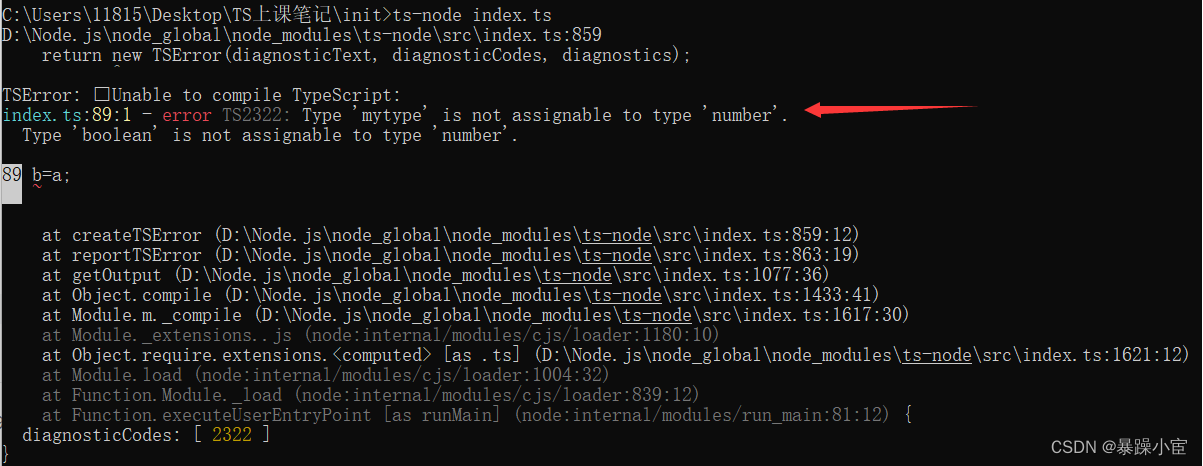``````type mytype = number|boolean;
function fn():mytype{
return 200
}
let a:mytype=fn();
let b:number=400;
b=(a as number); // 写入 as number
console.log(b);
``````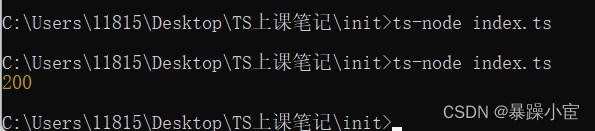### “TS基础了解一”的评论:

##### 关于作者##### overfit同步小助手